## 简明量子力学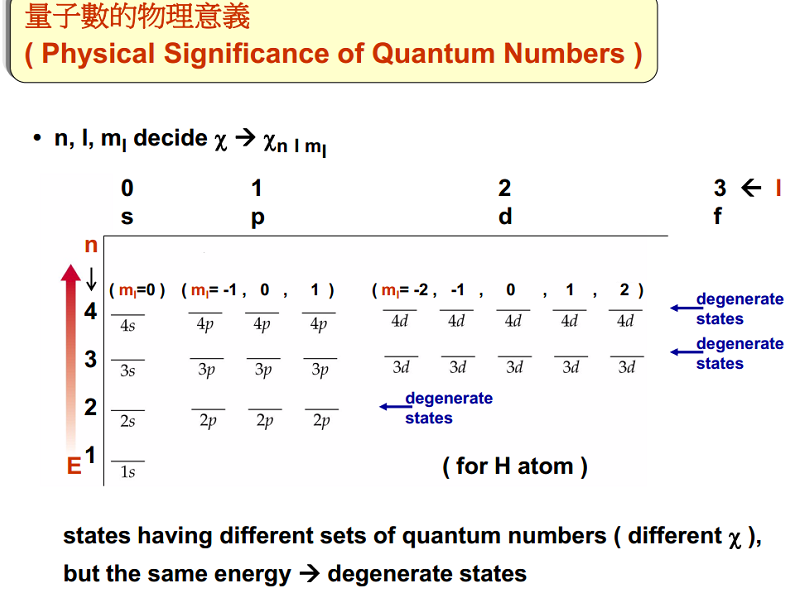1900年以前，经典物理学主要有四个问题不能解决(所以才引入量子论)：氢原子光谱的规律、光电效应、黑体辐射、原子的稳定性问题。

### 量子力学人物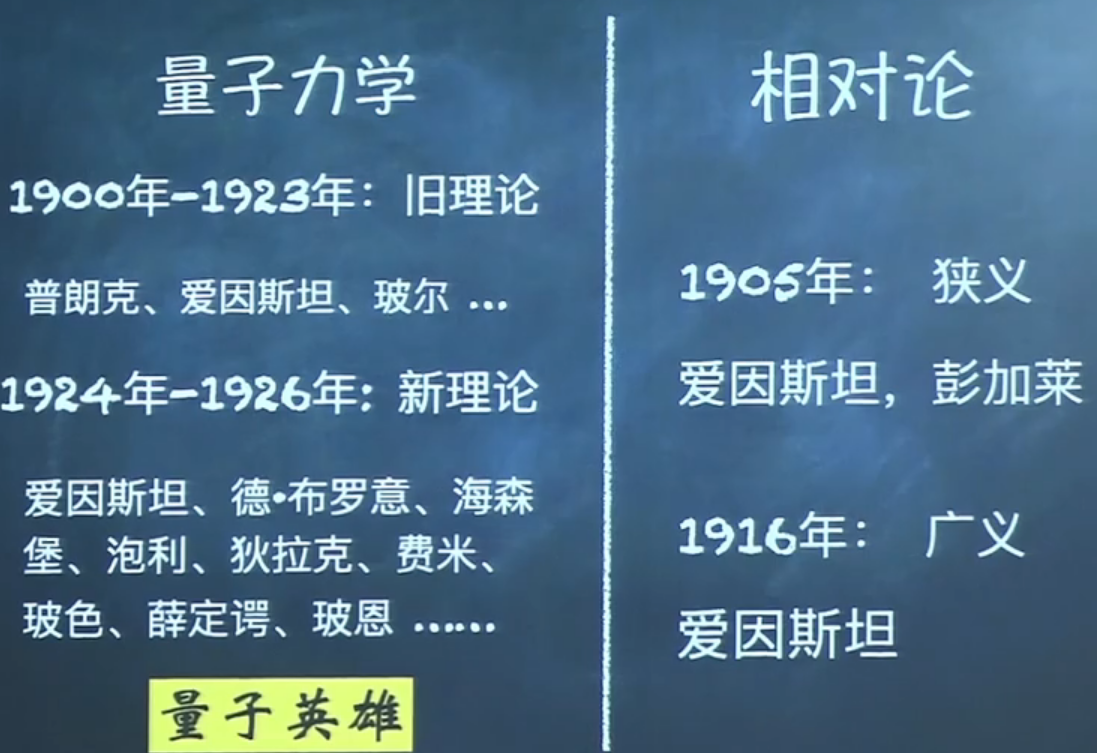(1) 光电效应：1905年，爱因斯坦看透了这个为人熟知的公式，发现了它后面隐藏的量子。通过和经典气体的熵类比，爱因斯坦发现黑体辐射可以看作是一种特殊的由 “光子”构成的气体，每个光子的能量是$$h \nu$$，“粒子和波的行为有本质的不同，光虽然被广泛认为是一种波，但在很多现象里，比如黑体辐射、荧光、和光致阴极辐射，光的行为更像粒子。”他用光量子的概念轻松地解释了光电效应。在当时的物理学 界，所有的人都认为光不是粒子，而是一种波——按照麦克斯韦方程振动和传播的电磁波。
(2) 固体比热：他将普朗克的黑体辐射公式应用固体比热。爱因斯坦认为固体中原子振动的能量也是一份一份的，它们应该同样遵守普朗克的黑体辐射公式。当时物理学家已经将温度降到摄氏−250度，他们在实验中发现固体比热会随着温度降低大幅减小。经典理论完全无法解释这个现象。爱因斯坦利用普朗克的公式发现，固体的比热确实会随温度降低而减小，而且自己推导出来的公式和已经发表的实验结果吻合得非常好。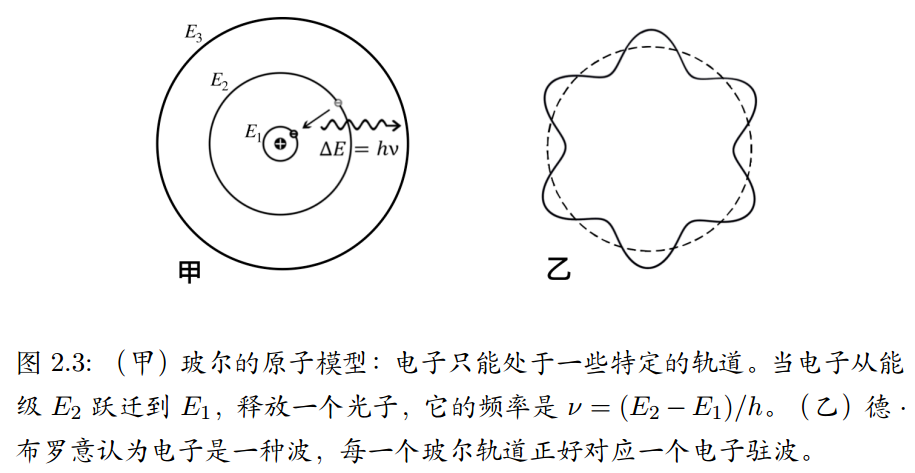(1) 索末菲是目前为止教导过最多诺贝尔物理学奖得主的人，他首先提出第二量子数(角量子数)和第四量子数(自旋量子数)，并且提出精细结构常数和开创了X射线波动理论。他学生中最有名的就是海森堡和泡利了，还有德拜(亚琛时期)，鲍林。
(2) 桃李满天下为大师之师，无缘于诺奖成无冕之王-张天蓉

(1) 他给爱因斯坦的信“我成功地推导出来了普朗克公式中的系数$$8 \pi \nu^{2} / c^{3}$$。在推导中没有用经典电动力学，而是假设相空间应该被分成很多小格每格大小是$$h^{3}$$。”
(2) 爱因斯坦立刻将波色全同这个概念推广，既然光子是全同的、不可区分，那么其他粒子也是一样的。爱因斯坦为此连续发表了三篇论文，在这些论文里爱因斯坦预言了著名的玻色-爱因斯坦凝聚现象。七十年后，1995年物理学家利用超冷原子气验证了爱因斯坦的预言。

20 岁时，海森堡勇敢地引入了半整量子数(在索末菲研讨班上提出的)；24岁时，海森堡勇敢地突破了旧的量子理论，创立了矩阵力学。

(1) 除了空间自由度外，电子还有有一个奇怪的自由度；
(2) 任何两个电子不能同时处于完全相同的量子态。

(1) 他1930年写的专著《量子力学原理》(The Principles of Quantum Mechanics) 时至今日依然没有过时，是每个物理系学生的必读。
(2) 他通过量子力学和狭义相对论结合，提出了一个新的波动方程，狄拉克方程，预言了反粒子的存在。
(3) 发现分析力学的泊松括号和海森堡矩阵力学的PQ-QP很像，于是基于此提出了正则量子化。

### 量子力学概念

(1) 一个量子系统的量子态可以用波函数来完全地表述。波函数代表一个观察者对于量子系统所知道的全部信息。
(2) 按照玻恩定则，量子系统的描述是概率性的。一个事件的概率是波函数的绝对值平方。(马克斯·玻恩)
(3) 不确定性原理阐明，在量子系统里，一个粒子的位置和动量无法同时被确定。(海森堡)
(4) 物质具有波粒二象性；根据互补原理，一个实验可以展示出物质的粒子行为，或波动行为；但不能同时展示出两种行为。(尼尔斯·玻尔)
(5) 测量仪器是经典仪器，只能测量经典性质，像位置，动量等等。
(6) 对应原理：大尺度宏观系统的量子物理行为应该近似于经典行为。(尼尔斯·玻尔与海森堡)

(1) 玻色子可以处于同样的量子态。光子、胶子、声子、与氦-4原子，都是玻色子。
(2) 费米子不能处于同样的量子态（这性质称为泡利不相容原理）。电子、中微子、夸克、质子、中子、氦-3原子，都是费米子。

#### 旧量子力学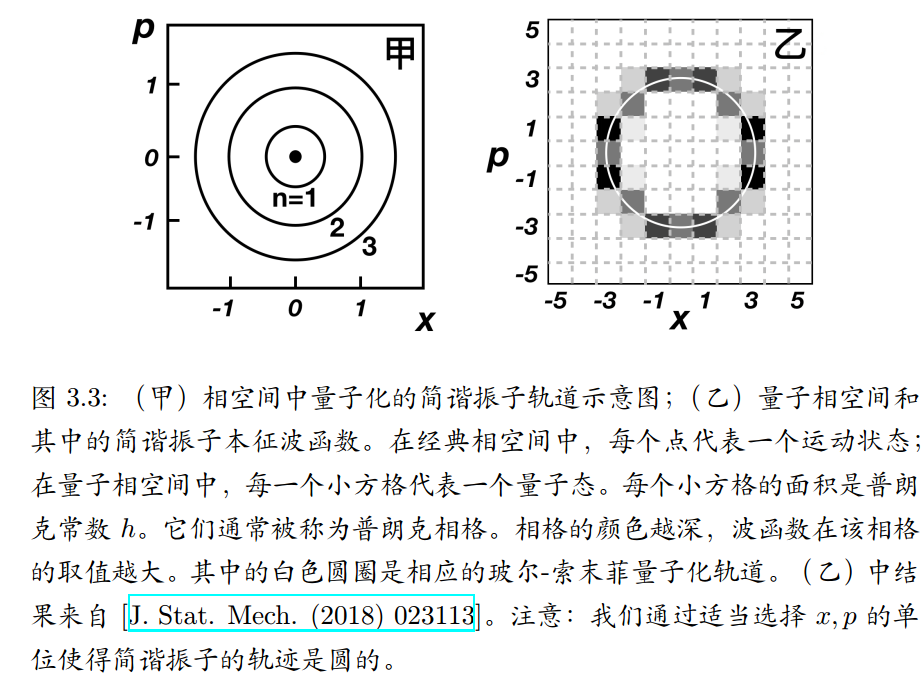玻尔和索末菲的量子化规则是：量子化轨道在相空间围出的面积$$S$$是普朗克常数$$h$$的整数倍，即$$S=\oint p d x=n h, \quad n=1,2,3, \cdots$$应用到简谐振子，对于一个给定的$$x_0$$其对应轨迹围成的面积是$$S=\pi p_{0} x_{0}=\pi m x_{0}^{2} \omega=2 \pi E / \omega$$这里$$E=m x_{0}^{2} \omega^{2} / 2$$是振子能量。如果这个$$x_0$$对应第$$n$$个量子化的能级$$E_n$$，那么我们应该有$$2 \pi E_{n} / \omega=n h$$ 我们于是得到量子化的简谐振子能级($$h=2 \pi \hbar$$)$$E_{n}=n \hbar \omega$$每个离散的能级$$E_n$$，在相空间都对应有一条经典轨迹。把玻尔和索末菲的量子化规则应用到氢原子就能得到氢原子的能级和量子化轨道。

### 线性代数汇总

(1) $$O$$一定有$$n$$  个本征态$$\left|\phi_{1}\right\rangle,\left|\phi_{2}\right\rangle, \cdots,\left|\phi_{n}\right\rangle$$, 其相应本征值为$$v_{1}, v_{2}, \cdots, v_{n}$$。 即$$O\left|\phi_{j}\right\rangle=v_{j}\left|\phi_{j}\right\rangle, \quad(j=1,2, \cdots, n)$$ 。
(2) 可以证明本征值$$v_{1}, v_{2}, \cdots, v_{n}$$都是实数。
(3) 可以证明本征态$$\left|\phi_{1}\right\rangle,\left|\phi_{2}\right\rangle, \cdots,\left|\phi_{n}\right\rangle$$ 相互正交，即$$\left\langle\phi_{i} \mid \phi_{j}\right\rangle=0,(i \neq j)$$。
(4) 由于$$c\left|v_{j}\right\rangle$$依然是本征态，我们可以利用这个性质，通过适当选择$$c$$使得$$\left\langle\phi_{j} \mid \phi_{j}\right\rangle=1$$。这种本征态被称作是归一的。
(5) 有可能某两个本征值$$v_{i}=v_{j}(i \neq j)$$，这说明相对应的两个本征态$$\left|\phi_{i}\right\rangle$$和$$\left|\phi_{j}\right\rangle$$简并。

### 迈入量子之门

#### 施特恩-格拉赫实验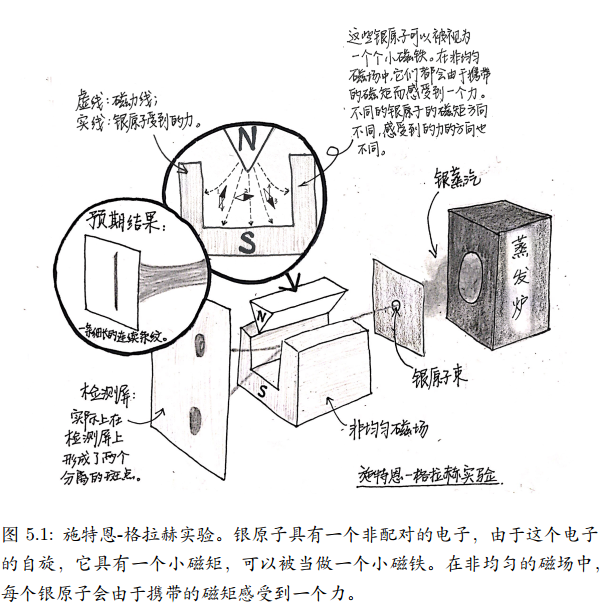1922年，两位德国物理学家的实验，高温炉将银加热蒸发成气体，银蒸汽从炉子里出来经过一些控制阀门形成银原子束，银原子束通过一个非均匀磁场后到达检测屏。他们发现银原子束会被非均匀磁场折射分解成两束，最后在检测屏上形成两个分离的斑点。这个结果完全出乎施特恩和格拉赫的预料。因为根据经典物理，他们预期观察到一条细长的连续条纹。

#### 自旋

• 自旋是微观粒子的一个内禀特征，和静止质量和电荷类似。但自旋的内涵要 丰富得多，因为它还是一种自由度(抽象空间的自由度，在经典力学中完全不存在)。
• 轨道角动量总是普朗克常数的整数倍$$m \hbar$$，$$m$$整数可正可负。
• 自旋对应的角动量则可以是$$\pm \hbar / 2, \pm \hbar, \pm 3 \hbar / 2$$。
• 自旋 1/2 指的就是这个自旋的最大角动量是$$\hbar / 2$$。质子和中子 的自旋也是 1/2。光子的自旋则是 1(实际取值只能是±1，不能取)，这意味着光子自旋的最大角动量是$$\hbar$$。
• 自旋还和费米子、玻色子紧密相关：费米子的自旋都是半整 数的，比如 1/2， 3/2， 5/2 等；玻色子的自旋都是整数的，比如 0， 1， 2 等。
• 狄拉克发现电子具有自旋 1/2 是狭义相对论和量子力 学结合的必然结果。

(1) 在量子力学中，自旋是粒子所具有的内禀性质，其运算规则类似于经典力学的角动量，并因此产生一个磁场。虽然有时会与经典力学中的自转（例如行星公转时同时进行的自转）相类比，但实际上本质是迥异的。经典概念中的自转，是物体对于其质心的旋转，比如地球每日的自转是顺着一个通过地心的极轴所作的转动。地球自转并不是内在性质。如果地球不转了,它还是地球,但是粒子如果自旋不一样,它就是一个新粒子。 粒子的自旋——是你不能想象的事情！